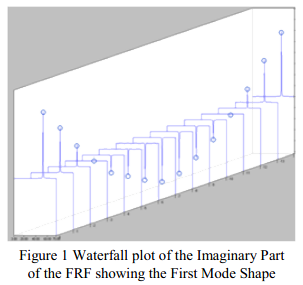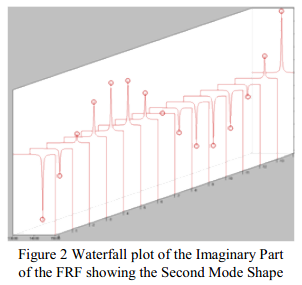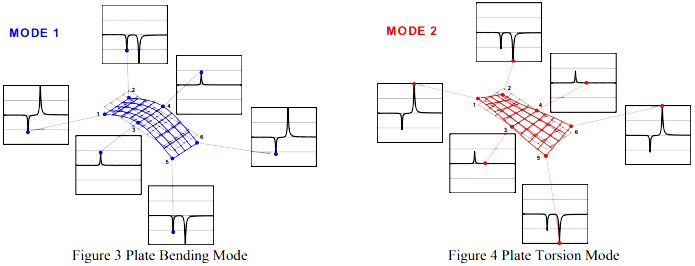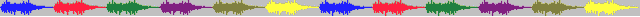# 95.模态空间13.10-如果力锤敲击一个节点，那么其他位置没有响应吗？

### 模态空间 – 在我们自己的小世界中     Pete Avitabile(著) KSI科尚仪器 董书伟（译）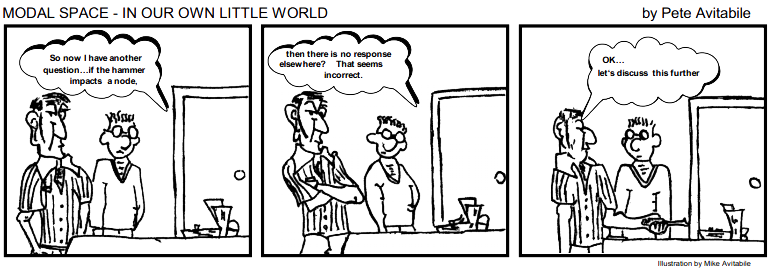#### 这看上去不对啊。好吧…我们深入讨论一下这个问题。

2014年05月05日 发布 ver1.0

$h\left ( s \right )_{ij}|_{s=j \omega}=h_{ij}\left ( j \omega \right )=\displaystyle\sum_{k=1}^{m}\dfrac{q_{k}u_{ik}u_{jk}}{\left ( j \omega-p_{k} \right )}+\dfrac{q_{k}^{*}u_{ik}^{*}u_{jk}^{*}}{\left (j \omega-p_{k}^{*} \right )}$